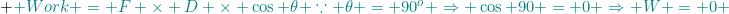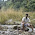## Sunday, 13 November 2011

### CBSE Class 9 - Chapter 11- Physics - Work And Energy (Q and A)

Work & Energy (Q & A)

Q1: A force of 7 N acts on an object. The displacement is, say 8 m, in the direction of the
force. Let us take it that the force acts on the object through the displacement. What
is the work done in this case?

Answer:  Let F be the force acting on the object displacing it by distance S, in the same direction of the force.
∴  F = 7N   and S = 8m
Work done (W) = Force (F) × Displacement (S)

= 7 x 8 = 56 Joules

Q2(NCERT): When do we say that work is done?

Answer: Work is said to be done when the following conditions are met:
(i) a force acts on the body.
(ii) A displacment of the body occurs by virtue of applied force in the direction of applied force.

Q3(NCERT): Define 1 J of work.

Answer: 1 J is the amount of work done by a force of 1 N on an object that displaces it through a distance of 1 m in the direction of the applied force.

Q4: Is Work a scalar or vector quantity?

Answer: Although work is product of two vectors, it is a scalar quantity.

Q5: Define 1 Watt.

Answer: When one-joule work is done in one second by any agent, then its power is said to be one watt. It is the unit of Power.

Q6: What is zero work?

Answer: When the force applied or the displacement is zero or when both are perpendicular to each other, zero work is done.

Mathematically,Q7: A stone of mass 2Kg is tied with a string and is whirled with a centripetal force of 10N in a circular path. How much work is done for making one revolution?

Answer: Zero work. Since, Centripetal Force (F) and the displacement direction are perpendicular to each other.

Q8: Calculate the amount of work done in drawing a bucket of water weighing 10kg from a well of depth 20m.

Answer: Given, mass m = 10 kg
Acceleration due to gravity,  g = 9.8 ms-2
Height h = 20 m

Here, work is done against gravity,
⇒ W = m.g.h = 10 × 9.8 × 20 = 1960 joules

Q9(NTSE): A box is pulled across a floor by applying a force of 100N  at an angle of 60 degrees above the horizontal. How much work is done by the applying force in pulling the object to a distance of 8m?

A force of 100 N is applied to a box of weight W. Force is a vector quantity applied at angle 60. It has two components, horizontal component (100 cos 60) and vertical component (100 sin 60).
The horizontal component of the force should be considered here, because it causes the displacement in horizontal direction.
∴ W = 100 cos 60 × 8 = 100 × 0.5 × 8 = 400 J

Q10: A body is thrown vertically upwards. Its velocity goes on decreasing. Write the change in kinetic energy when its velocity becomes zero.

Answer:  Zero KE = ½ mv2  and  v = 0

Q11: A force of 10 N moves a body with a constant speed of 2 m/s. Calculate the power of the body.

Q12: A boy of mass 45 kg climbs up 20 steps in 20 second. If each step is 25 cm high, calculate the power used in climbing

Q13: When a player hits a football it moves along the curved path and then falls to the ground. Calculate the work done by the force of gravity on the football.

Q14: Two friends Ram and Shyam, each having weight of 40 kg, go for rock climbing. Ram climbs to a height of 3 m in 10 s. and Shyam covers the same height in 12 s. Is the work done by Ram and Shyam equal ? or not ? Compare the power of Ram and Shyam.

Q15: Is it possible that a force acts on a body still the work done is zero? Explain with an example.

Answer: Yes. Consider a ball tied to a string is moving in a circular path. The centripetal force is  being applied on the ball and the centripetal acceleration is changing the direction every instant. Yet no work is done, since centripetal force acting upon is perpendicular to the displacement (of the moving ball).

Q16: Can a body have energy without possessing momentum?

Answer: Yes. Potential Energy is by virtue of height or position of the object. E.g. water in the tower tank has (potential) energy though at rest. Another example is compressed spring stores potential energy though it is at rest.

A nice presentation by an_empa (from Manila) on Work, Power and Energy.
Chapter 6 Work And Energy

Q17 (AIEEE): A pendulum of length 1m and with a bob of mass 1 kg swings in a vertical plane. Find the work done (from the below said choices) by weight of the bob when it moves from position A to B (as shown in figure). (Take g = 10 m/sec^2)
a. 4J
b. -4J
c. -5 J
d. 5 J

The work done by the bob (due to its weight) is change in PE by going up by height (h) vertically as shown by red arrow.

∴ h = l - l.cos60 = 1 - 1/2 = ½ metres
Gain in P.E. = mgh = 1 × 10  × ½ = -5J
The bob moves from lowest position to higher position, work done is negative.

Q18: Two bodies of masses m1 and m2  have equal kinetic energies.  If  p1 and p2 are their respective momentum, then ratio p1 : p2 is ___?

Answer: As given K.E.s are equal ½ m1v12  = ½ m2v22
∴ p1/ p2 = m1v1/ m2v2
⇒ p1/ p2 =  √m1/√m2

Q19: (AIEEE): An object of mass m is raised from the the earth to an height of equal to the radius of the earth (R). Calculate the gain in potential energy by the object. Assume gravity of the earth is g.

Note the Potential Energy formula i.e. PE = mgh cannot be applied here. This formula (PE = mgh) is a local approximation formula and is applicable when the height h is very small and near to the surface of the earth.
The gravitational potential energy at any point is given by the following formula:
U = -G(m1m2)/r
where, G = is Gravitational constant, m1and m2 are the masses of the two objects and r is the distance between them.

Gain in P.E. will be:
ΔUg = Ufinal - Uinitial =  -G(m1m2)/(r + Δh) + G(m1m2)/r

This comes out to be:
⇒ ΔUg = mgΔh. r / (r + Δh)

Applying it in our case.
Δh  = R
ΔUg = mgR2/ 2R = mgR/2         ... (answer)

Q20(NCERT): A pair of bullocks exerts a force of 140 N on a plough. The field being ploughed is 15 m long. How much work is done in ploughing the length of the field?

Answer: Given,  Force = 140 N
Distance covered = 15m
Work done = Force × Displacement
Force is applied in the direction of displacement.
⇒ Work done in ploughing = 140 × 15 = 2100 J
Thus 2100 joules of work is done in ploughing the field.

Q21(NCERT): What is the kinetic energy of an object?

Answer:Ability of a body to do work due to its motion is called kinetic energy.  Its SI unit is joules. Kinetic energy is directly proportional to the magnitude of velocity/speed. Higher the speed of an object, higher will be its kinetic energy.

Q22(NCERT): Write an expression for the kinetic energy of an object.

Answer: Let a body of mass m is moving with velocity v then its kinetic energy (K.E.) can be expressed as:
K.E. = ½ mv2
The SI unit of kinetic energy is joules.

DERIVATION: Let a body of mass m is lying stationary on a frictionless horizontal surface. When
a force F acts on it, in time t, it undergoes a displacement S, and it acquires velocity v

If  work done W during the process is expressed as:
W = Force × Displacement = F 2kgS

Since F = ma     where a is the acceleration.
⇒  W = maS                                                                ...(I)
From laws of motion we know that  v2- u2= 2aS
Since u = 0, ∴   v2 = 2aS
or aS = ½ mv2
Thus equation (I)   becomes,
W = ½ mv2

Q23(NCERT): The kinetic energy of an object of mass, m moving with a velocity of 5 m/s is 25 J. What will be its kinetic energy when its velocity is doubled? What will be its kinetic energy when its velocity is increased three times?

Answer: K.E. of the object = 25 J
velocity of the object (v) = 5 m/s
∵ K.E. = ½ mv2
⇒ m = 2 × K.E./v2
⇒ m = 2 × 25 / 25 = 2kg

if velocity is double, v = 2 × 5 = 10 m/s
∴ K.E. (for v = 10m/s) =   ½ mv2  = ½ × 2 × 100 = 100J

if velocity is tripled, v = 3 × 5 = 15 m/s
∴ K.E. (for v = 10m/s) =   ½ mv2  = ½ × 2 × 225 = 225J

Q 24(MCQ): A light and a heavy body have equal momenta. Which one has greater kinetic energy?

(a) The light body
(b) The heavy body
(c) Both have equal K.E.
(d) none of these

(In progress...)1.this site has really proved to be so much fruitfull to me

2.thanks for providing these solutions.................

3.simply good

4.5.Awesome site really helped me a lot.

6.Easy to understand...good results..😊 im satisfied

7.8.it made the study easier and more convenience

9.great job....easy to learn

10.is watt the SI unit of energy???????????????

11.